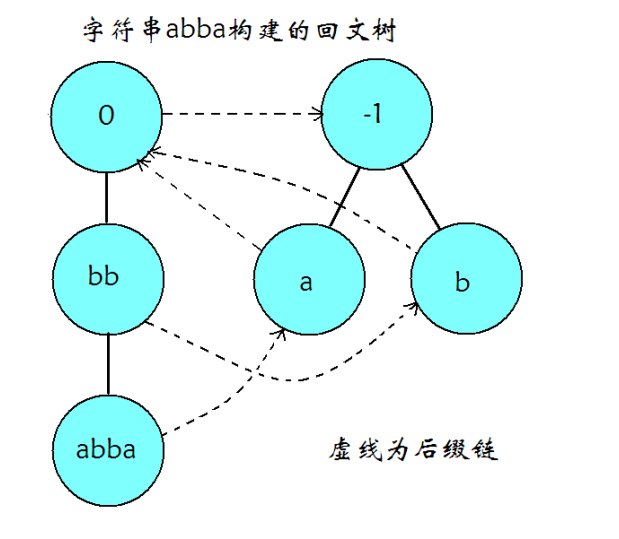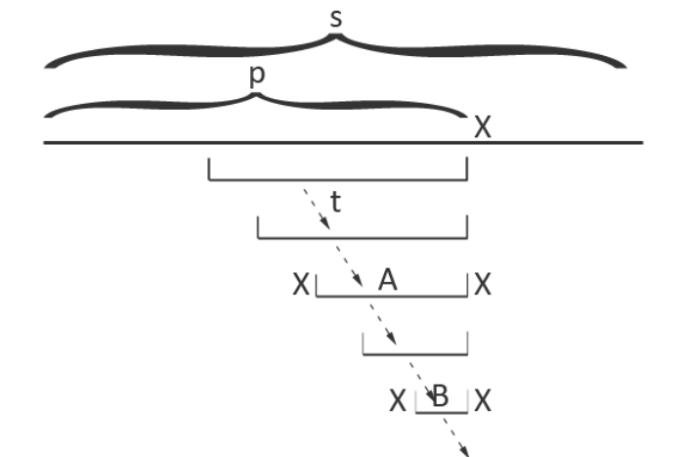# 回文树

## 结构¶## 建造¶## 线性状态数证明¶

• $|s| =1$ 时，$s$ 只有一个字符，同时也只有一个子串，并且这个子串是回文的，因此结论成立。

• $|s| >1$ 时，设 $t=sc$，其中 $t$ 表示 $s$ 最后增加一个字符 $c$ 后形成的字符串，假设结论对 $s$ 串成立。考虑以最后一个字符 $c$ 结尾的回文子串，假设它们的左端点由小到大排序为 $l_1,l_2,\dots,l_k$。由于 $t[l_1..|t|]$ 是回文串，因此对于所有位置 $l_1 \le p \le |t|$，有 $t[p..|t|]=t[l_1..l_1+|t|-p]$。所以，对于 $1 < i \le k$$t[l_i..|t|]$ 已经在 $t[1..|t|-1]$ 中出现过。因此，每次增加一个字符，本质不同的回文子串个数最多增加 $1$ 个。